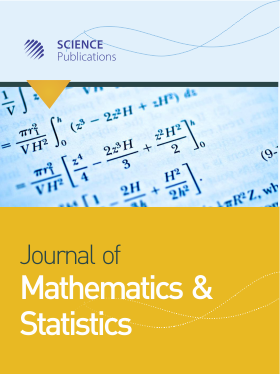Frequency: Continuous
ISSN: 1549-3644 (Print)
ISSN: 1558-6359 (Online)
Research Article Open Access

# On Gröbner Bases and Their Uses in Solving System of Polynomial Equations and Graph Coloring

Haridas Kumar Das1 and Nasim Reza1
• 1 University of Dhaka, Bangladesh
Journal of Mathematics and Statistics
Volume 14 No. 1, 2018, 175-182

DOI:

Published On: 27 June 2018

How to Cite: Das, H. K. & Reza, N. (2018). On Gröbner Bases and Their Uses in Solving System of Polynomial Equations and Graph Coloring. Journal of Mathematics and Statistics, 14(1), 175-182. https://doi.org/10.3844/jmssp.2018.175.182

## Abstract

This paper is based on the analytic and computational solution procedures of Gröbner basis and its applications. We show the behavior of the ideals generated by polynomials from a polynomial ring. We also present the idea of a zero dimensional ideal and use of this ideal to solve system of polynomial equations. We then introduce an algorithmic procedure for solving a system of polynomial equations (linear and nonlinear) with a finite number of solutions extending the idea of Gröbner basis. Finally we explore the idea of Gröbner basis for coloring the vertices of a given graph. We illustrate the stated results through a number of examples. Moreover, as for auxilary and making comparison with the analytic results, we use Mathematica 9.0.1 to develop some computer algebra.